### ATLAS_2017_I1591327

Back to index

A measurement of the production cross section for two isolated photons in proton--proton collisions at a center-of-mass energy of $\sqrt{s}=8$ TeV is presented. The results are based on an integrated luminosity of 20.2 fb$^{-1}$ recorded by the ATLAS detector at the Large Hadron Collider. The measurement considers photons with pseudorapidities satisfying $|\eta^\gamma| < 1.37$ or $1.56 < |\eta^\gamma| < 2.37$ and transverse energies of respectively $E^\gamma_\text{T,1}>40$ GeV and $E^\gamma_\text{T,2}> 30$ GeV for the two leading photons ordered in transverse energy produced in the interaction. The background due to hadronic jets and electrons is subtracted using data-driven techniques. The fiducial cross sections are corrected for detector effects and measured differentially as a function of six kinematic observables. The measured cross section integrated within the fiducial volume is $16.8\pm0.8$ pb. The data are compared to fixed-order QCD calculations at next-to-leading-order and next-to-next-to-leading-order accuracy as well as next-to-leading-order computations including resummation of initial-state gluon radiation at next-to-next-to-leading logarithm or matched to a parton shower, with relative uncertainties varying from 5% to 20%.

ATLAS_2017_I1591327_d02-x01-y01: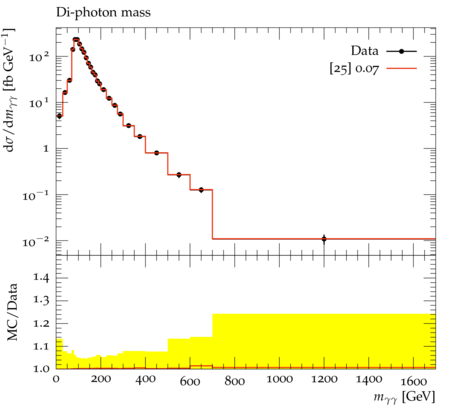ATLAS_2017_I1591327_d03-x01-y01: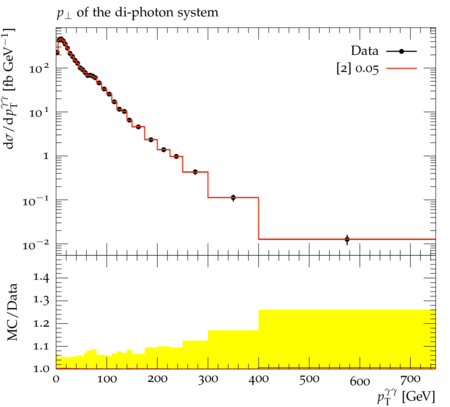ATLAS_2017_I1591327_d04-x01-y01: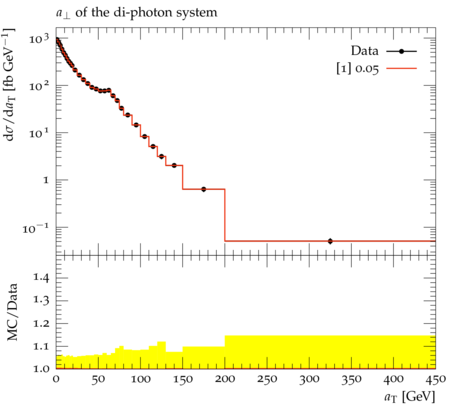ATLAS_2017_I1591327_d05-x01-y01: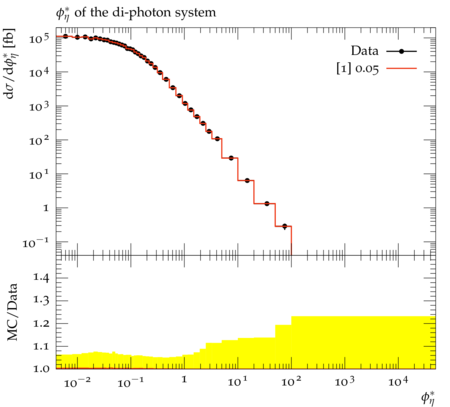ATLAS_2017_I1591327_d06-x01-y01: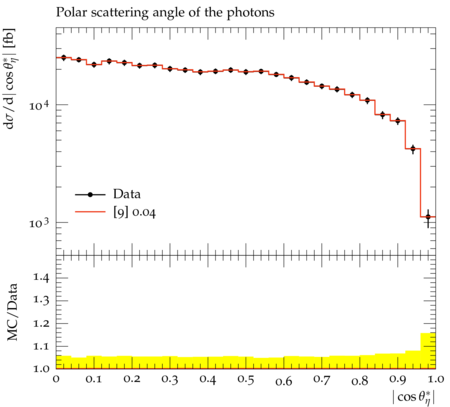ATLAS_2017_I1591327_d07-x01-y01: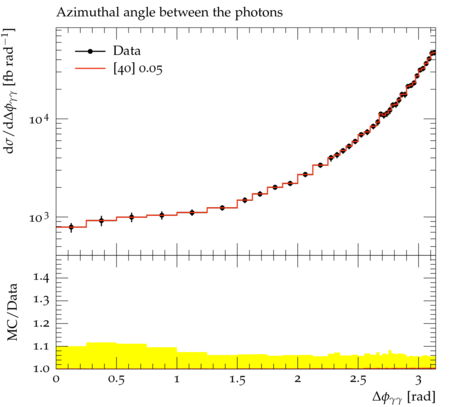Generated at Sunday, 28. January 2018 12:08PM